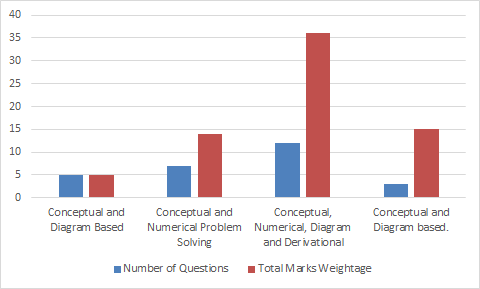# CBSE Class 12 Physics Paper Analysis 2019

CBSE Class 12 board exams have already started three days ago i.e.on 2nd of March. Students are done with their English paper, but the real challenge for Science stream students have just started with Physics paper conducted on 5th March 2019. According to the students who appeared for the Physics paper said that this year Physics paper was an average and a scoring paper compared to last year Physics paper. All the questions were from the syllabus as per the CBSE pattern and nothing came out of syllabus.

In terms of the difficulty level section D was slightly tough and challenging and Section C questions were somewhat tricky. Most of the questions asked in the Physics paper were numerical based due to which some students found the paper lengthy. As per the analysis 2 to 4 questions were almost similar to previous year Physics question paper.

The Physics question paper was divided into four sections Section A, B, C and D. More details given below:

 Section Number of questions Marks for each question Total marks A 5 1 5 B 7 2 14 C 12 3 36 D 3 5 15 Total 27 70

Section C contains the highest mark weightage which mainly consists of questions related to numerical problems, conceptual based and derivation based. The physics question paper was more conceptual than theoretical.

Type of Questions – Section-wise

 Major Type of Questions Number of Questions Total Marks Weightage Conceptual and Diagram Based 5 5 Conceptual and Numerical Problem Solving 7 14 Conceptual, Numerical, Diagram and Derivational 12 36 Conceptual and Diagram based. 3 15CBSE Class 12 Physics Question Paper Pattern

 Types of Question No of questions Question mark Total Very short answer question 5 1 5 Short Answer – I Question 7 2 14 Short Answer – I Question 12 3 36 Long answer type question 3 5 15 27 70

In conclusion, we can say that Class 12 Physics paper was average in terms of difficulty level. But in terms of time management, it might be an issue as the paper was a bit lengthy and tricky. Hope students of Class 12 have completed their paper on time so that they pass their Physics paper with flying colors.

 NCERT Related Articles NCERT Solutions for Class 12 NCERT Solutions for Class 11 NCERT Solutions for Class 10 NCERT Solutions for Class 12 Maths NCERT Solutions for Class 11 Maths NCERT Solutions for Class 10 Maths NCERT Solutions for Class 12 Physics NCERT Solutions for Class 11 Physics NCERT Solutions for Class 10 Science NCERT Solutions for Class 12 Chemistry NCERT Solutions for Class 11 Chemistry NCERT Solutions for Class 6 NCERT Solutions for Class 12 Biology NCERT Solutions for Class 11 Biology NCERT Solutions for Class 6 Maths NCERT Solutions for Class 9 NCERT Solutions for Class 8 NCERT Solutions for Class 6 Science NCERT Solutions for Class 9 Maths NCERT Solutions for Class 8 Maths NCERT Solutions for Class 4 NCERT Solutions for Class 9 Science NCERT Solutions for Class 8 Science NCERT Solutions for Class 4 Maths NCERT Solutions for Class 5 NCERT Solutions for Class 7 NCERT Solutions for Class 7 Maths NCERT Solutions for Class 4 Science NCERT Solutions for Class 5 Maths NCERT Solutions for Class 7 Science NCERT Solutions NCERT Books NCERT Solutions for Class 5 Science